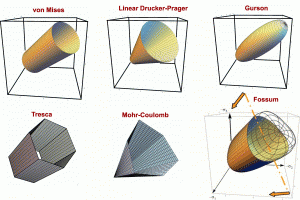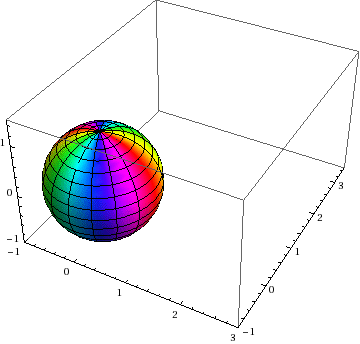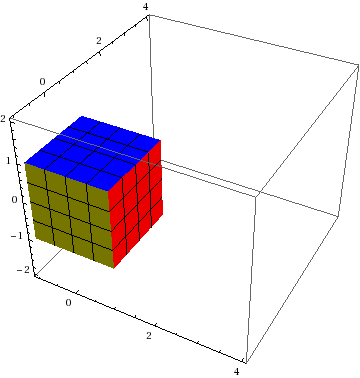# Hyphenation in technical (or other) writing.Should you say “finite element” or “finite-element?” Which is better: a “beautifully-written” manuscript, or “beautifully written” one? Are your equations non-linear or nonlinear? Our one-page list of hyphenation rules summarizes information found in a variety of authoritative sources (Princeton Review, Strunk & White, etc.). Happy technical writing!

# Illustration of polar decomposition

This posting explains the meaning of a polar decomposition, and it gives two numerical methods for computing it.

Below is shown simple shear of a unit square.  The inscribed circle and the lines from corner to corner should be regarded as painted on the material, so they flow with deformation.  The green and red dashed lines show the principal directions of stretch, which are aligned with the major axes of the deformed ellipse and hence move relative to the material as the deformation proceeds.  In the deformed state (far right), the red and green dashed lines are defined to be aligned with the major axes of the deformed ellipse (far right). The red and green dashed lines in the other states show the material points covered by those green and red lines in the deformed state.# Stretch, rotation, and intuition about strain rate approximations

The animation below shows
RED: simple shear in physical configuration
BLUE: simple shear with the polar rotation removed (i.e., the pure stretch)
GREEN: the deformation corresponding to the approximation that D-bar (given by the unrotated symmetric part of the velocity gradient) is actually the rate of reference logarithmic strain. This is found by integrating D-bar through time to obtain the apparent logarithmic strain, and then exponentiating this apparent strain to obtain an apparent reference stretch.
GRAY: rotation of the green deformation back to the spatial configuration.# Distinction between uniaxial stress and uniaxial strain

Uniaxial stress is a form of loading in which the 11 (axial) component of stress is nonzero, while all other components of stress are zero.

Uniaxial strain is a form of loading in which the 11 (axial) component of strain is nonzero, while all other components of strain are zero.

# Centroidal Voronoi Tesselations

The CSM lab at the University of Utah is actively developing the Material Point Method (MPM). Like other particle methods, the MPM discretizes a body into a set of points at which problem data (velocity, stress, temperature, etc.) are stored.  Centroidal Voronoi Tesselation is a promising way to distribute points within a domain in a way that is favorable to the MPM, where using a distribution of particles conforming to the boundary is highly desired.  For a thorough overview, see Max Gunzburger’s website,  http://people.sc.fsu.edu/~mgunzburger/cvt/cvt.html.  Some excerpts from his site are shown below,

# Comparison of Uniaxial Stress and Uniaxial Strain States

When doing verification of material models it is a very good idea to check both uniaxial stress and uniaxial strain states. For a von Mises material, we can analytically determine what displacements and stresses will be present when the material yields. The following table was created to aid those that work with the von Mises (or J2 plasticity) material model.

There are only three parameters that are used for the table; specifically, the yield stress in uniaxial tension and any two elastic modulii. The table was written so that if only Young’s modulus and Poisson’s ratio are known, the reader can derive any other required values by using the equations included at the bottom of the table.

The LaTeX source for the table can be found here.

# Publication: Elements of Phenomenological Plasticity: Geometrical Insight, Computational Algorithms, and Topics in Shock PhysicsThis 2007 Book Chapter on the basics of plasticity theory reviews the terminology and governing equations of plasticity, with emphasis on amending misconceptions, providing physical insights, and outlining computational algorithms. Plasticity theory is part of a larger class of material models in which a pronounced change in material response occurs when the stress (or strain) reaches a critical threshold level. If the stress state is subcritical, then the material is modeled by classical elasticity. The bound- ary of the subcritical (elastic) stress states is called the yield surface. Plasticity equations apply if continuing to apply elasticity theory would predict stress states that extend beyond this the yield surface. The onset of plasticity is typically characterized by a pronounced slope change in a stress–strain dia-gram, but load reversals in experiments are necessary to verify that the slope change is not merely nonlinear elasticity or reversible phase transformation.
The threshold yield surface can appear to be significantly affected by the loading rate, which has a dominant effect in shock physics applications.

In addition to providing a much-needed tutorial survey of the governing equations and their solution (defining Lode angle and other Lode invariants and addressing the surprisingly persistent myth that closest-point return satisfies the governing equations), this book chapter includes some distinctive contributions such as a simple 2d analog of plasticity that exhibits the same basic features of plasticity (such as existence of a “yield” surface with associative flow and vertex theory), an extended discussion of apparent nonassociativity, stability and uniqueness concerns about nonassociativity, and a  summary of apparent plastic wave speeds in relation to elastic wave speeds (especially noting that non-associativity admits plastic waves that travel faster than elastic waves).

For the full manuscript with errata, click 2007 Book Chapter on the basics of plasticity theory.

# Annulus Twist as a verification test

Illustrated below is the solution to an idealized problem of a linear elastic annulus (blue) subjected to twisting motion caused by rotating the T-bar an angle$\alpha$.  The motion is presumed to be applied slowly enough that equilibrium is satisfied.This simple problem is taken to be governed by the equations of equilibrium$\vec{\nabla}\cdot\sigma=0$, along with the plane strain version of Hooke’s law in which Cauchy stress is taken to be linear with respect to the small strain tensor (symmetric part of the displacement gradient).  If this system of governing equations is implemented in a code, the code will give you an answer, but it is up to you to decide if that answer is a reasonable approximation to reality. This observation helps to illustrate the distinction between verification (i.e., evidence that the equations are solved correctly) and validation (evidence that physically applicable and physically appropriate equations are being solved).  The governing equations always have a correct answer (verification), but that answer might not be very predictive of reality (validation).

# Exact solution for eigenvalues and eigenvectors/projectors of a real 3×3 symmetric matrixThe Pi (or Pie) Plane showing the region of the principal solution for the Lode angle and hence the region nearest the max eigenvalue.

It probably isn’t surprising that an exact solution can be found for eigenvalues of a real 3×3 symmetric matrix.  This conclusion follows from noting that the characteristic equation is cubic, for which an exact solution procedure can be found in any good algebra reference.  One thing that you won’t find in many such resources, however, is an algorithm for the solution that will avoid complex numbers in the intermediate steps of the calculation whenever the components of the source symmetric 3×3 matrix are all real.

# Tutorial: Visualizing Deformation

If you’ve never heard of a continuum mapping, read our introduction to mappings.

This posting discusses the two most common visualization methods for 3D homogeneous mappings: Showing how a sphere transforms to an ellipsoid and how a cube transforms to a parallelepiped: